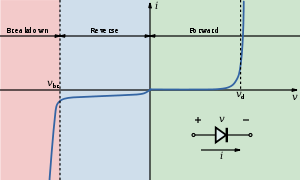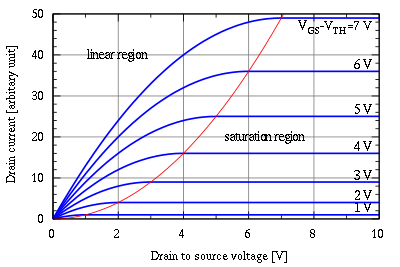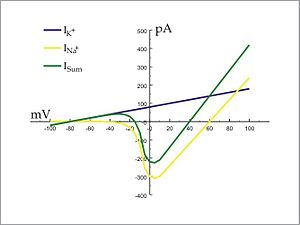# Current–voltage characteristic

Current–voltage characteristicFigure 1. I–V curve of a P–N junction diode (not to scale).

A current–voltage characteristic is a relationship, typically represented as a chart or graph, between an electric current and a corresponding voltage, or potential difference.

## In electronicsFigure 2. MOSFET drain current vs. drain-to-source voltage for several values of the overdrive voltage, VGSVth; the boundary between linear (Ohmic) and saturation (active) modes is indicated by the upward curving parabola

In electronics, the relationship between the direct current (DC) through an electronic device and the DC voltage across its terminals is called a current–voltage characteristic of the device. Electronic engineers use these charts to determine basic parameters of a device and to model its behavior in an electrical circuit. These characteristics are also known as I-V curves, referring to the standard symbols for current and voltage.

A more general form of current–voltage characteristic is one that describes the dependence of a terminal current on more than one terminal voltage difference; electronic devices such as vacuum tubes and transistors are described by such characteristics.

Figure 1 shows an I–V curve for a P-N junction diode. Figure 2 shows a family of I–V curves for a MOSFET as a function of drain voltage with overvoltage (VGS-Vth) as a parameter.

The simplest I–V characteristic involves a resistor, which according to Ohm's Law exhibits a linear relationship between the applied voltage and the resulting electric current. However, even in this case environmental factors such as temperature or material characteristics of the resistor can produce a non-linear curve.

The transconductance and Early voltage of a transistor are examples of parameters traditionally measured with the assistance of an I–V chart, or laboratory equipment that traces the charts in real time on an oscilloscope.

## In electrophysiologyFigure 3. An approximation of the potassium and sodium ion components of a so-called "whole cell" I–V curve of a neuron.

While I–V curves are applicable to any electrical system, they find wide use in the field of biological electricity, particularly in the sub-field of electrophysiology. In this case, the voltage refers to the voltage across a biological membrane, a membrane potential, and the current is the flow of charged ions across channels in this membrane. The current is determined by the conductances of these channels.

In the case of ionic current across biological membranes, currents are measured from inside to outside. That is, positive currents, known as "outward current", corresponding to positively charged ions crossing a cell membrane from the inside to the outside, or a negatively charged ion crossing from the outside to the inside. Similarly, currents with a negative value are referred to as "inward current", corresponding to positively charged ions crossing a cell membrane from the outside to the inside, or a negatively charged ion crossing from inside to outside

Figure 3 shows an I-V curve that is more relevant to the currents in excitable biological membranes (such as a neuronal axon). The blue line shows the I–V relationship for the potassium ion. Note that it is linear, indicating no voltage-dependent gating of the potassium ion channel. The yellow line shows the I–V relationship for the sodium ion. Note that it is not linear, indicating that the sodium ion channel is voltage-dependent. The green line indicates the I–V relationship derived from summing the sodium and potassium currents. This approximates the actual membrane potential and current relationship of a cell containing both types of channel.

Wikimedia Foundation. 2010.

### Look at other dictionaries:

• current-voltage characteristic — voltamperinė charakteristika statusas T sritis automatika atitikmenys: angl. current voltage characteristic; voltage current characteristic; volt ampere characteristic vok. Stromspannungscharakteristik, f; Strom Spannungs Kennlinie, f;… …   Automatikos terminų žodynas

• current-voltage characteristic — voltamperinė charakteristika statusas T sritis fizika atitikmenys: angl. current voltage characteristic; voltage ampere characteristic; volt ampere characteristic vok. Strom Spannungs Charakteristik, f; Strom Spannungskennlinie, f rus.… …   Fizikos terminų žodynas

• current-voltage characteristic — voltamperinė charakteristika statusas T sritis Standartizacija ir metrologija apibrėžtis Elektrinės grandinės elemento srovės stiprio priklausomybė nuo jį veikiančios įtampos. atitikmenys: angl. current voltage characteristic vok. Strom… …   Penkiakalbis aiškinamasis metrologijos terminų žodynas

• current-voltage characteristic — voltamperinė charakteristika statusas T sritis Standartizacija ir metrologija apibrėžtis Elektrinės grandinės elemento įtampos priklausomybė nuo juo tekančios srovės stiprio. atitikmenys: angl. current voltage characteristic vok. Strom… …   Penkiakalbis aiškinamasis metrologijos terminų žodynas

• current-voltage characteristic meter — voltamperinės charakteristikos matuoklis statusas T sritis radioelektronika atitikmenys: angl. current voltage characteristic meter; current voltage curve meter vok. Strom Spannungs Kennlinie Messer, m rus. измеритель вольт амперной… …   Radioelektronikos terminų žodynas

• nonsaturation current-voltage characteristic — neįsotintoji voltamperinė charakteristika statusas T sritis radioelektronika atitikmenys: angl. nonsaturation current voltage characteristic vok. Strom Spannungs Charakteristik ohne Sätingungsabschnit, f rus. вольт амперная характеристика без… …   Radioelektronikos terminų žodynas

• current-voltage curve meter — voltamperinės charakteristikos matuoklis statusas T sritis radioelektronika atitikmenys: angl. current voltage characteristic meter; current voltage curve meter vok. Strom Spannungs Kennlinie Messer, m rus. измеритель вольт амперной… …   Radioelektronikos terminų žodynas

• Characteristic — (from the Greek word for a property or attribute (= trait) of an entity) may refer to: In physics and engineering, any characteristic curve that shows the relationship between certain input and output parameters, for example: I V or current… …   Wikipedia

• voltage-current characteristic — voltamperinė charakteristika statusas T sritis automatika atitikmenys: angl. current voltage characteristic; voltage current characteristic; volt ampere characteristic vok. Stromspannungscharakteristik, f; Strom Spannungs Kennlinie, f;… …   Automatikos terminų žodynas

• voltage-ampere characteristic — voltamperinė charakteristika statusas T sritis fizika atitikmenys: angl. current voltage characteristic; voltage ampere characteristic; volt ampere characteristic vok. Strom Spannungs Charakteristik, f; Strom Spannungskennlinie, f rus.… …   Fizikos terminų žodynas